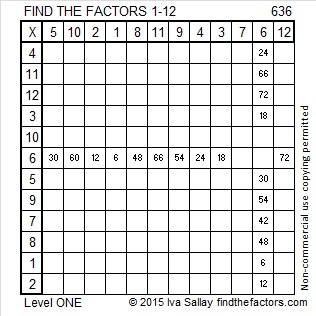# 636 and Level 1

636 is the sum of the ten prime numbers from 43 to 83.

636 is also the hypotenuse of the Pythagorean triple 336-540-636. What is the greatest common factor of those three numbers?Print the puzzles or type the solution on this excel file: 12 Factors 2015-10-05

—————————————————————————————————

• 636 is a composite number.
• Prime factorization: 636 = 2 x 2 x 3 x 53, which can be written 636 = (2^2) x 3 x 53
• The exponents in the prime factorization are 2, 1, and 1. Adding one to each and multiplying we get (2 + 1)(1 + 1)(1 + 1) = 3 x 2 x 2 = 12. Therefore 636 has exactly 12 factors.
• Factors of 636: 1, 2, 3, 4, 6, 12, 53, 106, 159, 212, 318, 636
• Factor pairs: 636 = 1 x 636, 2 x 318, 3 x 212, 4 x 159, 6 x 106, or 12 x 53
• Taking the factor pair with the largest square number factor, we get √636 = (√4)(√159) = 2√159 ≈ 25.21904—————————————————————————————————